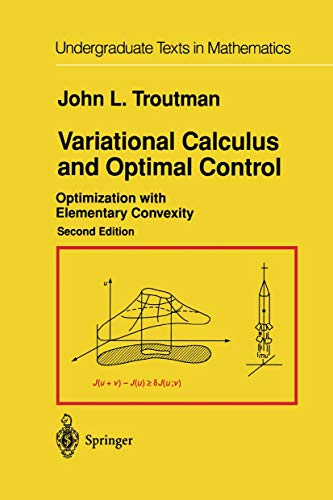• Free Shipping on all orders in Australia
• Over 7 million books in stock
• Proud to be B-Corp
• We aim to be carbon neutral by 2022
• Over 120,000 Trustpilot reviews
Item 1 of 0# Variational Calculus and Optimal Control by John L. Troutman

Condition - New
\$119.99
Only 2 left

## Summary

An introduction to the variational methods used to formulate and solve mathematical and physical problems, allowing the reader an insight into the systematic use of elementary (partial) convexity of differentiable functions in Euclidian space.

## Variational Calculus and Optimal Control Summary

### Variational Calculus and Optimal Control: Optimization with Elementary Convexity by John L. Troutman

An introduction to the variational methods used to formulate and solve mathematical and physical problems, allowing the reader an insight into the systematic use of elementary (partial) convexity of differentiable functions in Euclidian space. By helping students directly characterize the solutions for many minimization problems, the text serves as a prelude to the field theory for sufficiency, laying as it does the groundwork for further explorations in mathematics, physics, mechanical and electrical engineering, as well as computer science.

0 Review of Optimization in ?d.- Problems.- One Basic Theory.- 1 Standard Optimization Problems.- 1.1. Geodesic Problems.- (a) Geodesics in ?d.- (b) Geodesics on a Sphere.- (c) Other Geodesic Problems.- 1.2. Time-of-Transit Problems.- (a) The Brachistochrone.- (b) Steering and Control Problems.- 1.3. Isoperimetric Problems.- 1.4. Surface Area Problems.- (a) Minimal Surface of Revolution.- (b) Minimal Area Problem.- (c) Plateau's Problem.- 1.5. Summary: Plan of the Text.- Notation: Uses and Abuses.- Problems.- 2 Linear Spaces and Gateaux Variations.- 2.1. Real Linear Spaces.- 2.2. Functions from Linear Spaces.- 2.3. Fundamentals of Optimization.- Constraints.- Rotating Fluid Column.- 2.4. The Gateaux Variations.- Problems.- 3 Minimization of Convex Functions.- 3.1. Convex Functions.- 3.2. Convex Integral Functions.- Free End-Point Problems.- 3.3. [Strongly] Convex Functions.- 3.4. Applications.- (a) Geodesics on a Cylinder.- (b) A Brachistochrone.- (c) A Profile of Minimum Drag.- (d) An Economics Problem.- (e) Minimal Area Problem.- 3.5. Minimization with Convex Constraints.- The Hanging Cable.- Optimal Performance.- 3.6. Summary: Minimizing Procedures.- Problems.- 4 The Lemmas of Lagrange and Du Bois-Reymond.- Problems.- 5 Local Extrema in Normed Linear Spaces.- 5.1. Norms for Linear Spaces.- 5.2. Normed Linear Spaces: Convergence and Compactness.- 5.3. Continuity.- 5.4. (Local) Extremal Points.- 5.5. Necessary Conditions: Admissible Directions.- 5.6*. Affine Approximation: The Frechet Derivative.- Tangency.- 5.7. Extrema with Constraints: Lagrangian Multipliers.- Problems.- 6 The Euler-Lagrange Equations.- 6.1. The First Equation: Stationary Functions.- 6.2. Special Cases of the First Equation.- (a) When f = f(z).- (b) When f = f(x,z).- (c) When f = f(y,z).- 6.3. The Second Equation.- 6.4. Variable End Point Problems: Natural Boundary Conditions.- Jakob Bernoulli's Brachistochrone.- Transversal Conditions*.- 6.5. Integral Constraints: Lagrangian Multipliers.- 6.6. Integrals Involving Higher Derivatives.- Buckling of a Column under Compressive Load.- 6.7. Vector Valued Stationary Functions.- The Isoperimetric Problem.- Lagrangian Constraints*.- Geodesics on a Surface.- 6.8*. Invariance of Stationarity.- 6.9. Multidimensional Integrals.- Minimal Area Problem.- Natural Boundary Conditions.- Problems.- Two Advanced Topics.- 7 Piecewise C1 Extremal Functions.- 7.1. Piecewise C1 Functions.- (a) Smoothing.- (b) Norms for ?1.- 7.2. Integral Functions on ?1.- 7.3. Extremals in ?1 [a, b]: The Weierstrass-Erdmann Corner Conditions.- A Sturm-Liouville Problem.- 7.4. Minimization Through Convexity.- Internal Constraints.- 7.5. Piecewise C1 Vector-Valued Extremals.- Minimal Surface of Revolution.- Hilbert's Differentiability Criterion*.- 7.6*. Conditions Necessary for a Local Minimum.- (a) The Weierstrass Condition.- (b) The Legendre Condition.- Bolza's Problem.- Problems.- 8 Variational Principles in Mechanics.- 8.1. The Action Integral.- 8.2. Hamilton's Principle: Generalized Coordinates.- Bernoulli's Principle of Static Equilibrium.- 8.3. The Total Energy.- Spring-Mass-Pendulum System.- 8.4. The Canonical Equations.- 8.5. Integrals of Motion in Special Cases.- Jacobi's Principle of Least Action.- Symmetry and Invariance.- 8.6. Parametric Equations of Motion.7*. The Hamilton-Jacobi Equation.- 8.8. Saddle Functions and Convexity; Complementary Inequalities.- The Cycloid Is the Brachistochrone.- Dido's Problem.- 8.9. Continuous Media.- (a) Taut String.- The Nonuniform String.- (b) Stretched Membrane.- Static Equilibrium of (Nonplanar) Membrane.- Problems.- 9 Sufficient Conditions for a Minimum.- 9.1. The Weierstrass Method.- 9.2. [Strict] Convexity of f(x,Y, Z).- 9.3. Fields.- Exact Fields and the Hamilton-Jacobi Equation*.- 9.4. Hilbert's Invariant Integral.- The Brachistochrone*.- Variable End-Point Problems.- 9.5. Minimization with Constraints.- The Wirtinger Inequality.- 9.6*. Central Fields.- Smooth Minimal Surface of Revolution.- 9.7. Construction of Central Fields with Given Trajectory: The Jacobi Condition.- 9.8. Sufficient Conditions for a Local Minimum.- (a) Pointwise Results.- Hamilton's Principle.- (b) Trajectory Results.- 9.9*. Necessity of the Jacobi Condition.- 9.10. Concluding Remarks.- Problems.- Three Optimal Control.- 10 Control Problems and Sufficiency Considerations.- 10.1. Mathematical Formulation and Terminology.- 10.2. Sample Problems.- (a) Some Easy Problems.- (b) A Bolza Problem.- (c) Optimal Time of Transit.- (d) A Rocket Propulsion Problem.- (e) A Resource Allocation Problem.- (f) Excitation of an Oscillator.- (g) Time-Optimal Solution by Steepest Descent.- 10.3. Sufficient Conditions Through Convexity.- Linear State-Quadratic Performance Problem.- 10.4. Separate Convexity and the Minimum Principle.- Problems.- 11 Necessary Conditions for Optimality.- 11.1. Necessity of the Minimum Principle.- (a) Effects of Control Variations.- (b) Autonomous Fixed Interval Problems.- Oscillator Energy Problem.- (c) General Control Problems.- 11.2. Linear Time-Optimal Problems.- Problem Statement.- A Free Space Docking Problem.- 11.3. General Lagrangian Constraints.- (a) Control Sets Described by Lagrangian Inequalities.- (b)* Variational Problems with Lagrangian Constraints.- (c) Extensions.- Problems.- A.1. The Intermediate and Mean Value Theorems.- A.2. The Fundamental Theorem of Calculus.- A.3. Partial Integrals: Leibniz' Formula.- A.4. An Open Mapping Theorem.- A.5. Families of Solutions to a System of Differential Equations.- A.6. The Rayleigh Ratio.- Historical References.- Answers to Selected Problems.

NPB9781461268871
9781461268871
1461268877
Variational Calculus and Optimal Control: Optimization with Elementary Convexity by John L. Troutman
New
Paperback
Springer-Verlag New York Inc.
2012-09-30
462
N/A
Book picture is for illustrative purposes only, actual binding, cover or edition may vary.
This is a new book - be the first to read this copy. With untouched pages and a perfect binding, your brand new copy is ready to be opened for the first time